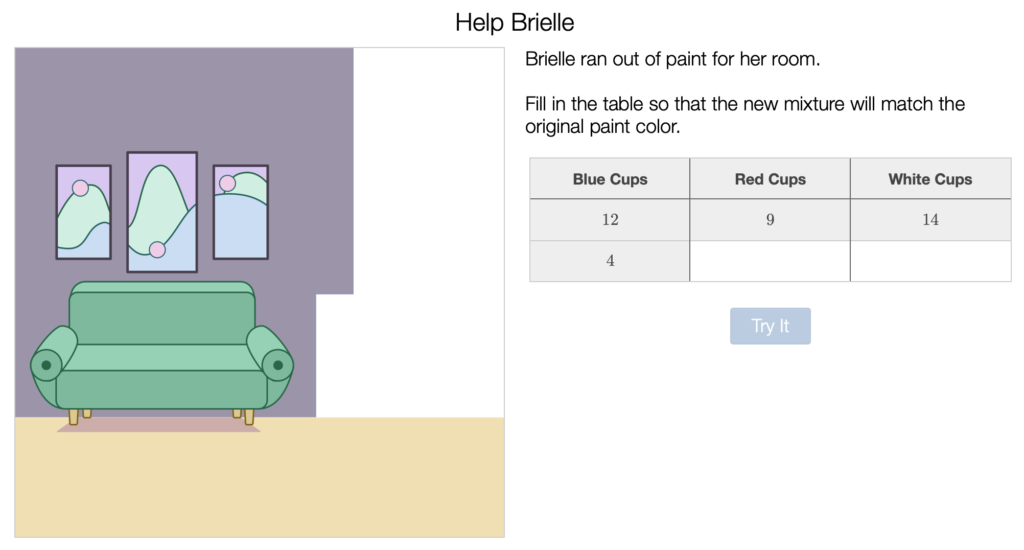# Desmos Reviews for Ratios, Proportions, Rates, Percent

Here are some Desmos activities that focus on Ratios, Proportions, Rates and Percent and my comments and reviews.

## Paint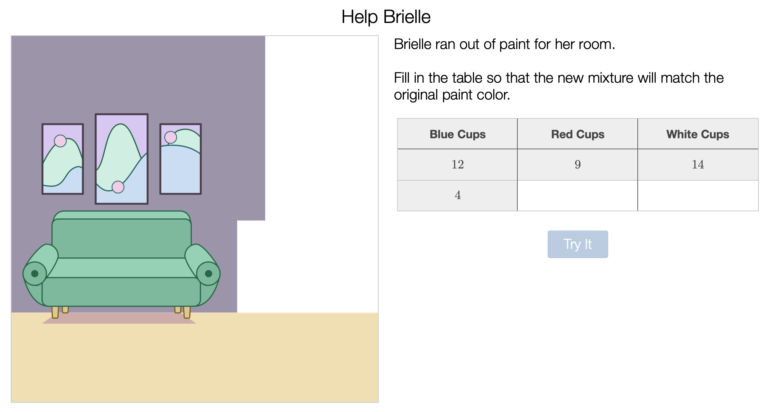Students use up to four colors to mix colors and complete the wall.  If the paint matches, they are successful.

In the example above, the mix is 12 cups of blue, 9 cups of red and 14 cups of white to create the color of the wall behind the couch.  The challenge is to start with 4 cups of blue and create the same color.

There are five warm up slides and then they proceed to class challenges where they use four colors to create their own.

I like this problem and would ask them to explain their answers to get to an understanding of ratios.

## Click Battle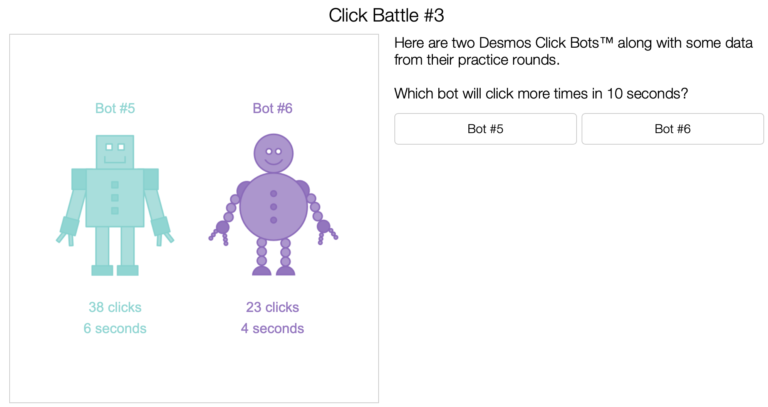Click Battle does not have any actual battles.  As you can see in the slide, students select which bot will click more times in 10 seconds.  There are 14 total slides with six battles and then a final battle.

This is rather simple.  After they select their answer, they are asked to explain their reasoning.  This may be where the best thinking can be accomplished. I had one student claim that Bot #5 would click more because his arms are closer to his body.

In the early slides, some may have used unit rate without realizing.  I would have a discussion around this in groups to guide them towards the concept of unit rate before introducing it.  In slide 8, unit rate is introduced.

## Des-Farm

This is essentially a 100 square with plants.  Students use the 100 square to play with Ratios, Fractions, Decimals and Percents.

This is convenient, but I would spend some time doing Concrete work with manipulatives prior to this activity since it is Representational and then Abstract.

If they have trouble or get stuck, it may be time to get out a concrete manipulative.

## Balloon Float

This one is closer to concrete.  You choose the number of balloons and then test to see if it floats.  Students will use ratios to determine the number of balloons.

There is one slide that asks students to model with linear equations.  Here it is: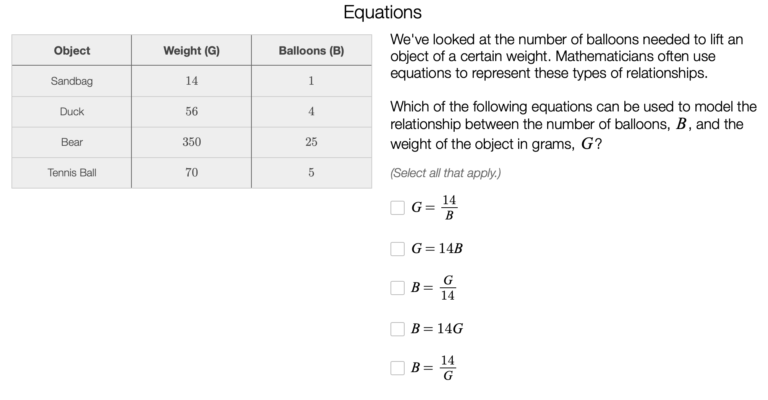## Nana's Chocolate Milk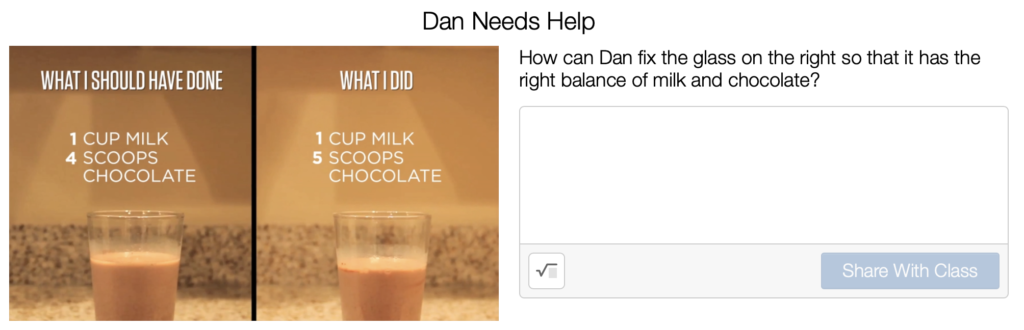This DESMOS activity is based on a Dan Meyer three-Act task, which you can see by clicking the button above.  It begins with a video of Dan putting too many scoops of chocolate in the Milk.  It is a lesson on using a double number line to get the correct ratio.

I actually prefer the original three-act task because it is more open and allows for better group work.  If you use the DESMOS activity, you may want to use the pacing tool to stop them after the first slide to give them time to think before going beyond the video.  You could even have them standing at vertical white boards in small groups.

## Exchange Rates

The student exchanges between Aloha, Beta and Gamma coins.  This is a lesson in ratios and also linear equations.  The ratios are represented as a table, graph and equations.

## Water Slide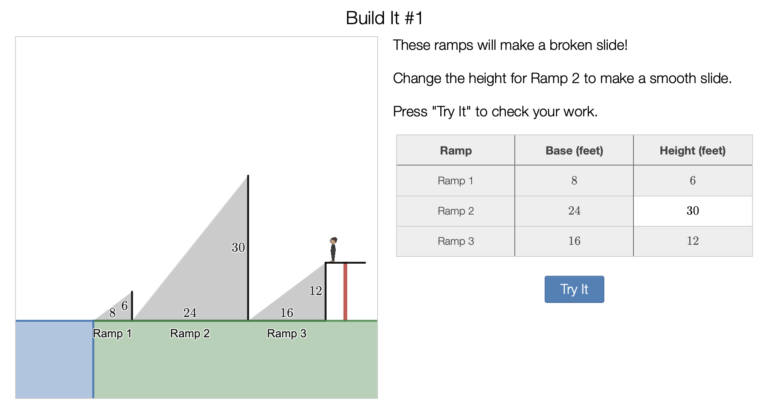Students adjust the ratio of height to width to create slides with the same slope.  This lesson could be use to give a concrete example of slope.  It is a very simple short example of making equal slopes.  It could be used as an early activity in Algebra or later in Pre-Algebra.